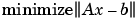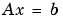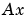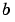MATLAB Function Referenceqr

Orthogonal-triangular decomposition

Syntax

• ```[Q,R] = qr(A)      (full and sparse matrices)
[Q,R] = qr(A,0)    (full and sparse matrices)
[Q,R,E] = qr(A)    (full matrices)
[Q,R,E] = qr(A,0)  (full matrices)
X = qr(A)          (full matrices)
R = qr(A)          (sparse matrices)
[C,R] = qr(A,B)    (sparse matrices)
R = qr(A,0)        (sparse matrices)
[C,R] = qr(A,B,0)  (sparse matrices)
```

Description

The `qr` function performs the orthogonal-triangular decomposition of a matrix. This factorization is useful for both square and rectangular matrices. It expresses the matrix as the product of a real orthonormal or complex unitary matrix and an upper triangular matrix.

```[Q,R] = qr(A) ``` produces an upper triangular matrix `R` of the same dimension as `A` and a unitary matrix `Q` so that `A` `=` `Q*R`. For sparse matrices, `Q` is often nearly full. If `[m n] = size(A)`, then `Q` is `m`-by-`m` and `R` is `m`-by-`n`.

```[Q,R] = qr(A,0) ``` produces an "economy-size" decomposition. If `[m n] = size(A)`, and `m > n`, then `qr` computes only the first `n` columns of of `Q` and `R` is `n`-by-`n`. If `m <= n`, it is the same as `[Q,R] = qr(A)`.

```[Q,R,E] = qr(A) ``` for full matrix `A`, produces a permutation matrix `E`, an upper triangular matrix `R` with decreasing diagonal elements, and a unitary matrix `Q` so that `A*E = Q*R`. The column permutation `E` is chosen so that `abs(diag(R))` is decreasing.

```[Q,R,E] = qr(A,0) ``` for full matrix `A`, produces an "economy-size" decomposition in which `E` is a permutation vector, so that `A(:,E) = Q*R`. The column permutation `E` is chosen so that `abs(diag(R))` is decreasing.

```X = qr(A) ``` for full matrix `A`, returns the output of the LAPACK subroutine `DGEQRF` or `ZGEQRF`. `triu(qr(A))` is `R`.

```R = qr(A) ``` for sparse matrix `A`, produces only an upper triangular matrix, `R`. The matrix `R` provides a Cholesky factorization for the matrix associated with the normal equations,

• ```R'*R = A'*A
```

This approach avoids the loss of numerical information inherent in the computation of `A'*A`. It may be preferred to `[Q,R] = qr(A)` since `Q` is always nearly full.

```[C,R] = qr(A,B) ``` for sparse matrix `A`, applies the orthogonal transformations to `B`, producing `C = Q'*B` without computing `Q`. `B` and `A` must have the same number of rows.

```R = qr(A,0) and [C,R] = qr(A,B,0) ``` for sparse matrix `A`, produce "economy-size" results.

For sparse matrices, the Q-less QR factorization allows the solution of sparse least squares problems

•with two steps

• ```[C,R] = qr(A,b)
x = R\c
```

If `A` is sparse but not square, MATLAB uses the two steps above for the linear equation solving backslash operator, i.e., `x = A\b`.

Examples

• ```A =  [ 1     2     3
4     5     6
7     8     9
10    11    12 ]
```

This is a rank-deficient matrix; the middle column is the average of the other two columns. The rank deficiency is revealed by the factorization:

• ```[Q,R] = qr(A)

Q =

-0.0776    -0.8331     0.5444     0.0605
-0.3105    -0.4512    -0.7709     0.3251
-0.5433    -0.0694    -0.0913    -0.8317
-0.7762     0.3124     0.3178     0.4461

R =

-12.8841    -14.5916    -16.2992
0     -1.0413     -2.0826
0           0      0.0000
0           0           0
```

The triangular structure of `R` gives it zeros below the diagonal; the zero on the diagonal in `R(3,3)` implies that `R`, and consequently `A`, does not have full rank.

Example 2. This examples uses matrix `A` from the first example. The QR factorization is used to solve linear systems with more equations than unknowns. For example, let

• ```b = [1;3;5;7]
```

The linear systemrepresents four equations in only three unknowns. The best solution in a least squares sense is computed by

• ```x = A\b
```

which produces

• ```Warning: Rank deficient, rank = 2, tol = 1.4594E-014
x =
0.5000
0
0.1667
```

The quantity `tol` is a tolerance used to decide if a diagonal element of `R` is negligible. If `[Q,R,E]` `=` `qr(A)`, then

• ````tol` `=` `max(size(A))`*`eps`*```abs(R(1,1))
``````

The solution `x` was computed using the factorization and the two steps

• ```y = Q`'`*b;
x = R\y
```

The computed solution can be checked by forming. This equalsto within roundoff error, which indicates that even though the simultaneous equationsare overdetermined and rank deficient, they happen to be consistent. There are infinitely many solution vectors `x`; the QR factorization has found just one of them.

Algorithm

The `qr` function uses LAPACK routines to compute the QR decomposition:

 Syntax Real Complex `R = qr(A)R = qr(A,0)` `DGEQRF` `ZGEQRF` `[Q,R] = qr(A)[Q,R] = qr(A,0)` `DGEQRF`, `DORGQR` `ZGEQRF`, `ZUNGQR` [Q,R,e] = qr(A)[Q,R,e] = qr(A,0) `DGEQP3`, `DORGQR` `ZGEQPF`, `ZUNGQR`

`lu`, `null`, `orth`, `qrdelete`, `qrinsert`, `qrupdate`
The arithmetic operators `\` and `/`qmr qrdelete